Courses

# Flow Measurement Civil Engineering (CE) Notes | EduRev

## Civil Engineering (CE) : Flow Measurement Civil Engineering (CE) Notes | EduRev

The document Flow Measurement Civil Engineering (CE) Notes | EduRev is a part of the Civil Engineering (CE) Course Civil Engineering SSC JE (Technical).
All you need of Civil Engineering (CE) at this link: Civil Engineering (CE)

Chapter 6 Flow Measurement

 Device Measurement Venturimeter rate of flow (discharge) Flow nozzle rate of flow Orifice meter rate of flow Bend meter rate of flow Rotameter rate of flow Pitot tube velocity Hot wire anemometer air & gas velocity Current meter velocity in open channels

Venturimeter:
-Cone angle of convergence side (20°) is greater than cone angle of divergence side (5° to 7°).
-Position of venturimeter does not play any role in discharge thus it is constant for horizontal, inclined or vertical position.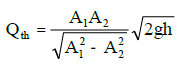-Qac = Cd Qth
-Cd = coefficient of discharge = 0.95 to 0.98
-Throat diameter is known as size of the venturimenter.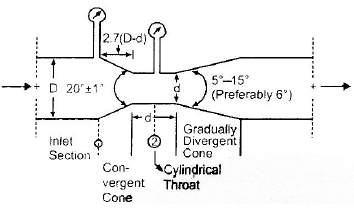

Orifice meter:
-It is a cheaper arrangement but has more energy loss.
-Cd for orifice meter = 0.68 - 0.74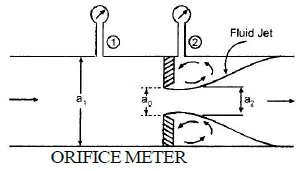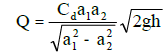-a1 = πd2/4
-a2 = πdc2/4

Pitot Tube:
-It is based on principle of conversion of kinetic head into pressure head. The point at which velocity reduces zero is called stagnation point.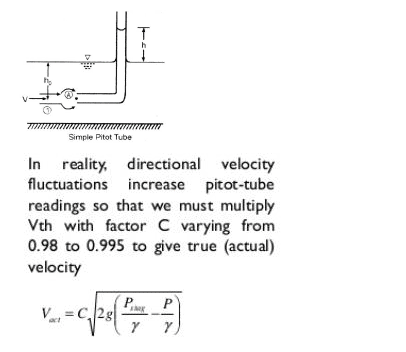-Vac = Cv .Vth
-Cv = Coefficient of velocity = 0.99
-ps/γ = stagnation head &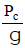static head.
-Velocity head is indicated by the difference in liquid level between the Pitot tube and the piezometer.The Pitot tube measures the total head and therefore known as total head tube.

Hydraulic Coefficients:
- Contraction coefficient (Cc) =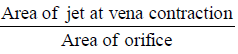- Coefficient of velocity of (Cv) =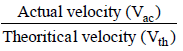- Coefficient of discharge (Cd) =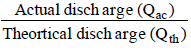- Cd = Cv . Cc

Flow through orifice in the tank: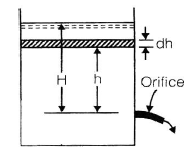Discharge through orifice when head over orifice is h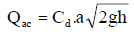Time required to lower the level from H to h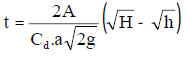Rectangular Weir/Notch: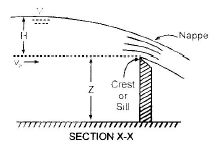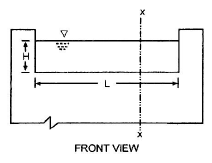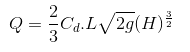L = length of crest
H = head above crest 

Triangular weir: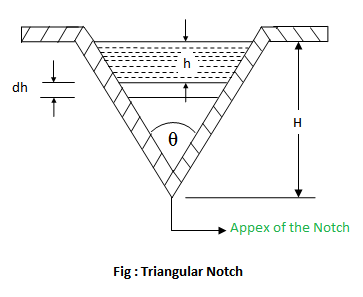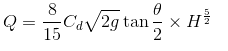Flow over cippoletti weir or trapezoidal weir:
-Trapezoidal weir is also called as Cippoletti weir. This is trapezoidal in shape and is the modification of rectangular weir with slightly higher capacity for same crest strength.
-
The sides are inclined outwards with a slope 1:4 (horizontal : vertical)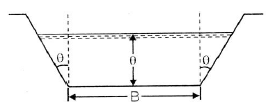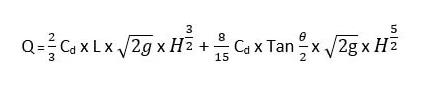Offer running on EduRev: Apply code STAYHOME200 to get INR 200 off on our premium plan EduRev Infinity!

## Civil Engineering SSC JE (Technical)

109 docs|50 tests

,

,

,

,

,

,

,

,

,

,

,

,

,

,

,

,

,

,

,

,

,

;